# MP Board Class 6th Maths Solutions Chapter 5 Understanding Elementary Shapes Ex 5.4

## MP Board Class 6th Maths Solutions Chapter 5 Understanding Elementary Shapes Ex 5.4

Question 1.
What is the measure of
(i) a right angle?
(ii) a straight angle?
Solution: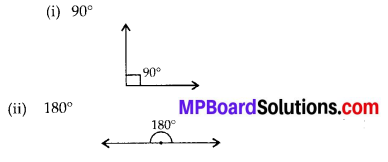Question 2.
Say True or False:
(a) The measure of an acute angle < 90°.
(b) The measure of an obtuse angle < 90°.
(c) The measure of a reflex angle > 180°.
(d) The measure of one complete revolution = 360°.
(e) If m∠A = 53° and m∠B = 35°, then m∠A > m∠B.
Solution:
(a) True
(b) False
Since, measure of an obtuse angle is greater than 90° and less than 180°.
(c) True
(d) True
(e) TrueQuestion 3.
Write down the measures of
(a) some acute angles.
(b) some obtuse angles.
(give at least two examples of each).
Solution:
(a) Measures of 2 acute angles are 35°, 20°
(b) Measures of 2 obtuse angles are 110°, 135°

Question 4.
Measure the angles given below using the Protractor and write down the measure.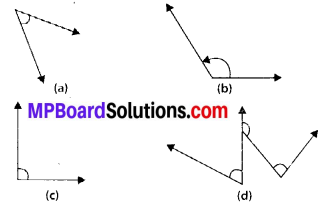Solution:
(d) Near about 60°, 120°, 90°
Note: Students can measure the angles exactly with the help of protractor.Question 5.
Which angle has a large measure? First estimate and then measure.
Measure of Angle A =
Measure of Angle B =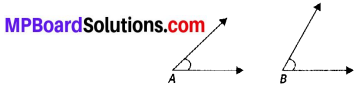Solution:
By estimating, we observe that ∠B has a large measure.
65°.
Note: Students can measure the angles exactly with the help of protractor.

Question 6.
From these two angles which has larger measure? Estimate and then confirm by measuring them.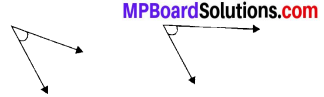Solution:
Second angle has larger measure.
First angle is near about 30° and second angle is near about 70°.
Note: Students can measure the angles exactly with the help of protractor.Question 7.
Fill in the blanks with acute, obtuse, right or straight:
(a) An angle whose measure is less than that of a right angle is ___.
(b) An angle whose measure is greater than that of a right angle is ___.
(c) An angle whose measure is the sum of the measures of two right angles is ___.
(d) When the sum of the measures of two angles is that of a right angle, then each one of them is ___.
(e) When the sum of the measures of two angles is that of a straight angle and if one of them is acute then the other should be ___.
Solution:
(a) Acute
(b) Obtuse
(c) Straight
(d) Acute
(e) Obtuse

Question 8.
Find the measure of the angle shown in each figure. (First estimate with your eyes and then find the actual measure with a protractor).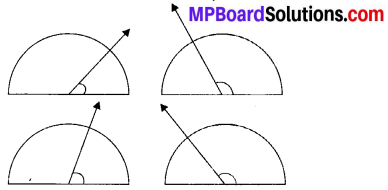Solution:
(i) By estimating with our eyes, we came to know that the measure of angle is
(ii) By estimating with our eyes, we came to know that the measure of angle is 120°.
(iii) By estimating with our eyes, we came to know that the measure of angle is 60°.
(iv) By estimating with our eyes, we came to know that the measure of angle is 150°.
Note: Students can measure the angles exactly with the help of protractor.Question 9.
Find the angle measure between the hands of the clock in each figure: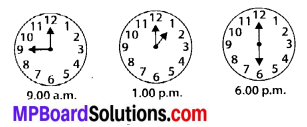Solution:
(i) 90° (Right angle)
(ii) 30° (Acute angle)
(iii) 180° (Straight angle)

Question 10.
Investigate
In the given figure, the angle measures 30°. Look at the same figure through a magnifying glass. Does the angle becomes larger? Does the size of the angle change?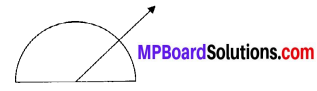Solution:
No, the measure of angle will be same.Question 11.
Measure and classify each angle: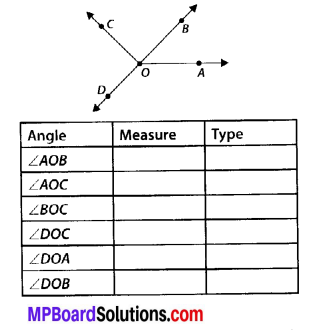Solution: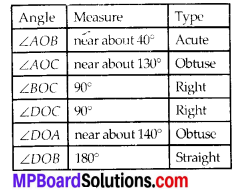Note: Students can measure the angles exactly with the help of protractor.